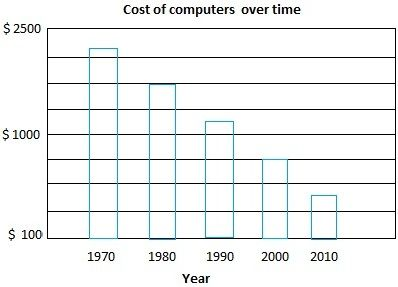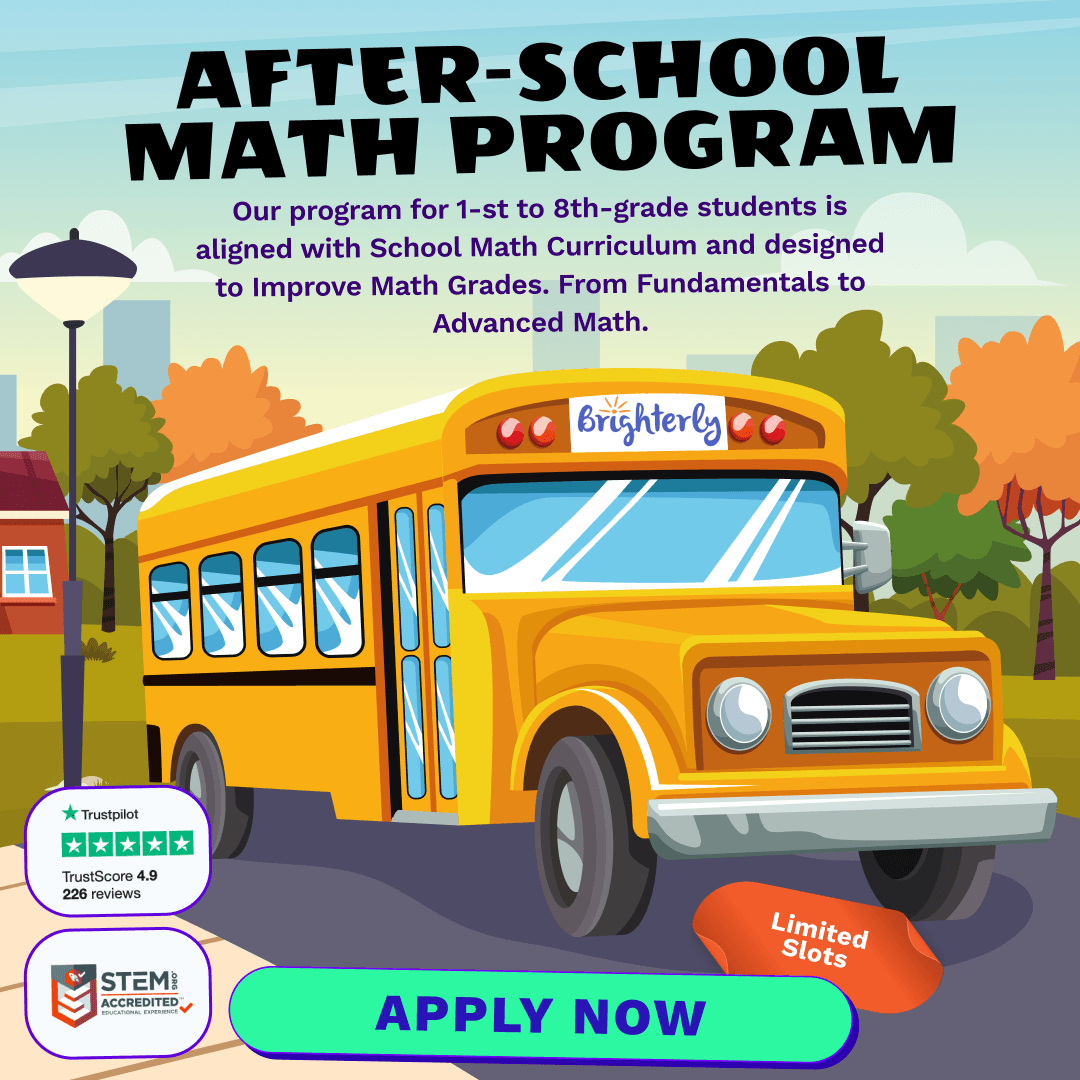# 5th Grade Diagnostic Math Test

Math TestsIt’s no longer news that many kids get cold feet when preparing to take math tests. But even when they’re confident, there’s still a chance they can perform below expectations.

Luckily, there are a few ways to assess your child’s level of preparation, and one of them is to let them take a diagnostic test. Read on to learn about the benefits of a diagnostic test and the possibility to download 5th grade math assessment tests for your child.

## The Importance and Benefits of Diagnostic 5th Grade Math Tests

Below are some of the benefits your child gains when they take the 5th grade math pre test:

### Growing Confidence

One of the reasons your child is dreading their math test is that they are not confident they can pass. But with the 5th grade math test and answers, they get to practice and correct their errors before they take the real tests. The diagnostic test is not graded. Hence, kids are more relaxed while taking it. This approach can help build their confidence and relieve much pressure as they prepare to take the real assessments.

### Assessing Math Skills

You’re never too sure of your abilities until you’re tested, and the same is true for kids preparing to take a math test. Your child may think they are ready, but they’ll never know how truly prepared they are until they see the test questions. This is why a diagnostic test is crucial. With this test, you can evaluate the readiness for a real test or the need to study more.

### Improving Learning Speed

The math benchmark test 5th grade is not just for students preparing to take a test. A teacher may also use it to check a student’s math skills to determine their strengths and weaknesses. This way, a teacher can tweak the lessons to lay more emphasis on areas where a student needs to improve.

Let’s say your child takes the diagnostic math test and correctly answers questions on factorization but cannot provide correct answers on geometry questions. This shows that your child understands the concept of factorization but isn’t too familiar with geometry. With this information, a teacher can adjust math lessons and focus more on geometry while spending less time learning math. This approach helps speed up a child’s learning.

Solve the following problems:

1. Find the value of “?” in the equation below

(4 + 2) × 5 = ? + 25

2. Jane’s special purse can hold 20 lipsticks. If given 440 lipsticks, how many purses will Jane need to hold them?
1. 21
2. 23
3. 20
4. 22
3. Illustrate the operation listed below with an integer. Then place them in the correct order.

A gain of 60 dollars ___________

A debit of 10 dollars___________

10 feet above sea level____________

A loss of 15 dollars ____________

_________, ___________, ____________, ______________

4. Try to put your finger on the edge of a CD, then move it all around the edge until it arrives at the same start-off point. You have calculated the _______________ of the CD

1. Surface area
2. Volume
3. Circumference
4. Area
5. Jack, Judie, and Sarah studied for 5 hours, 9 hours, and 7 hours respectively. Find the average number of hours the kids were studying?

1. 8
2. 7
3. 5
4. 8
6. Calculate:

-4 + -8 = _______

4 + 8 = _______

-4 + 8 = ______

4 + -8 = ______

7. Using <, >, or =, compare the decimals below

470.1550789           470.1550791

8. Calculate the area and perimeter of the rectangle given the following information: the rectangle’s length is 7, and width is 5.

Perimeter = _____________ units

Area = __________________ square units

9. If you toss a quarter, the possible outcomes are the following:__________________________

What is the probability of getting heads as a decimal ____________

What is the probability of getting heads as a fraction ____________

What is the probability of getting heads as a percent ____________

10. How much is 2/3 + 5/6?
11. What is the answer for number 10 as a mixed number _________________

What is the answer for number 10 in lowest terms _________________

12. Divide 7860 by 15 ________________
13. At Mr. Parker’s ice cream place, you can buy rum raisin, strawberry, peanut butter, and vanilla-flavored ice creams. He offers chocolate-coated cones, cake cones, and sugar cones.

a) Draw a tree below to represent various combinations of ice cream and cones that can be ordered at Mr. Parker’s ice cream place.

b) How many orders is it possible to make?

1. 3
2. 12
3. 4
4. 7
14. What do you have to do to find the number on the right?

4  19

2  16

7  34

12  61

1. Multiply the left number by 4 and then add 3
2. Multiply the left number by 3 and then add 10
3. Multiply the left number by 5 and then add 1
4. Multiply the left number by 5 and then subtract 1
15. The best way to measure the book’s length is in

1. Centimeters
2. Kilos
3. Millimeters
4. Kilometers
16. What is “?” in the questions below?

64837 -? = 62930

59 + ? = 29 + 36

17. If the length of the sides of an isosceles triangle are 6 and 10, what is the perimeter of the shape?

1. 22 or 23
2. 26 or 22
3. 26 or 21
4. 12 or 20
18. Given the following situations, what information could you identify?

Noemy is left with \$2.35 after buying a box of apples for \$4.20.

1. Noemy’s monthly allowance
2. The cost of apples
3. The amount of money the girl had before she bought the fruit
4. The number of apples in the box
19. Carefully study the following graph and then select the right answer1. In 2010, computers will cost half the price in comparison to their cost in 1970.
2. The cost of a computer decreased by more than 2000 dollars.
3. You can get a computer for 50 dollars in 2020.
4. There may be no charges for computers In 2030.
20. You have 1000 dollars and you want to distribute them between you and 5 of your friends.

1. Will the money be shared evenly?
2. How much maximum can be shared evenly?
3. Share the leftover, and define the amount each person gets as a fraction.

Note: If your child correctly answers 15 or more questions, it’s an indication that they have mastered most of the topics taught in the 5th grade. But if your child scores below 15, it means they have not fully mastered the topics taught in the 5th grade and that they need more help to improve their math skills.

## Printable 5th Grade Math Assessment Test

If you liked the 5th grade math questions above and would like to get more practice questions for your child, below are links to 5th grade end of year math test pdf files. You can download the 5th grade math assessment test printable files so your child can get more materials to practice.

## What Math Skills Are Required to Take a 5th Grade Math Test?

To take the 5th grade math test, your child needs to be proficient in the following areas:

• Operations: Students who want to take the printable 5th grade math test should be able to solve problems in multiplication, addition, subtraction, and division.
• Algebraic Thinking: Students who take the 5th grade made test should be able to write algebraic expressions, solve expressions with one variable, define variables, and solve algebraic equations.
• Geometry: The test of math for 5th grade also includes questions in geometry, like how to find the area of shapes like rectangles and triangles. They should also be able to find the areas of volumes.
• Ratios: Students in the fifth grade who want to take the diagnostic test should also use their knowledge of operations to solve world problems using ratios. They should also solve unit rate problems.

## Look Online for More Math Help

If your child does not perform well in the 5th grade math tests online, it means they need more help to improve their math skills. Luckily, a few websites offer online math classes for kids. One such website is Brighterly.com.

Brighterly provides one-on-one private math classes for students to help them improve their math skills. This platform has experienced tutors who can help take your child’s math skills from where they currently are to where they need to be. Brighterly uses interactive classes with fun-based learning methods to make the lessons enjoyable and effective. With the diagnostic test, Brighterly will assess your child’s strengths and weaknesses to help them structure their lessons for maximum effect.

After-School Math Programs• Our program for 1st to 8th grade students is aligned with School Math Curriculum.

After-School Math Programs
Our program for 1st to 8th grade students is aligned with School Math Curriculum.